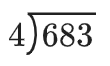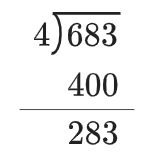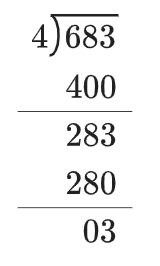### Home > CC1 > Chapter 4 > Lesson 4.1.1 > Problem4-10

4-10.

Read the Math Notes box in this lesson. Then complete the following division problems.

1. $683 \div 4$

1. $212 \div 9$

• Each step will be shown below. A division box shows 4 on the left, and 683 inside.

•  In the division box, now 400 is below 683, with difference of 283. Think ''$4$ goes into $600$ how many times?''
At least $100$ times, but not more than $200$.

• In the division box, now 280 is below 283, with difference of 3.  Think ''$4$ goes into $280$ how many times?''
Exactly $70$ times.
Think ''$4$ goes into $3$ how many times?''
Exactly $0.75$ times.1. $212 \div 9$

• Follow the steps outlined in part (a).